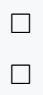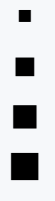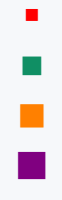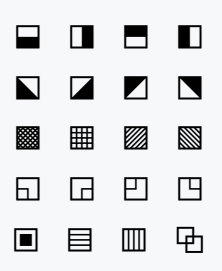# How to type square(□) symbol in LaTeX?

Square symbols are required at paragraph level or in different cases. This symbol has no default commands.

However, \square and \Box Command will not show the output in the document without the amssymb package.

\documentclass{article}
\usepackage{amssymb}
\begin{document}
$\Box$ % use for \Box command
$\square$ % use for \square command
\end{document}

Output :Above was the Empty Square symbol. If you need a filled symbol, it would be best practice to implement a stix package in the document.

Because there are different varieties of sizes in this package.

\documentclass{article}
\usepackage{stix}
\begin{document}
$\smblksquare$
$\mdblksquare$
$\mdlgblksquare$
$\lgblksquare$
\end{document}

Output :And if you think “I want different colors”. Of course, that is very easily possible in latex.

\documentclass{article}
\usepackage{stix}
\definecolor{boxcolor}{HTML}{108f64}
\begin{document}
${\color{red}\smblksquare}$
% Use HTML color code
${\color{boxcolor}\mdblksquare}$
${\color{orange}\mdlgblksquare}$
${\color{violet}\lgblksquare}$
\end{document}

Output :## stix package used for different styles of square symbols

Stix packages contain a variety of styled symbols. Which we always use in documents. You may have noticed that this symbol is either filled with a dot symbol or half-filled.

There are also many styles which are shown in the following output.

\documentclass{article}
\usepackage{stix}
\begin{document}
$\squarebotblack\quad \squarerightblack\quad \squaretopblack\quad \squareleftblack$
$\squarellblack\quad \squarelrblack\quad \squareulblack\quad \squareurblack$
$\squarecrossfill\quad \squarehvfill\quad \squareneswfill\quad \squarenwsefill$
$\squarellquad\quad \squarelrquad\quad \squareulquad\quad \squareurquad$
$\blackinwhitesquare\quad \squarehfill\quad \squarevfill\quad \boxonbox$
\end{document}

Output :#### Md Jidan Mondal

LaTeX expert with over 10 years of experience in document preparation and typesetting. Specializes in creating professional documents, reports, and presentations using LaTeX.